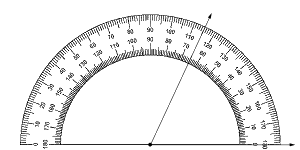• ### Browse All Lessons

##### Assign Lesson

Help Teaching subscribers can assign lessons to their students to review online!

 Tweet# Measuring Angles with ProtractorsIntroduction: An angle forms when two lines or rays join at a point called a vertex. If you try to measure the distance between those two rays with a ruler, you will find that the measure will be smaller closer to the vertex and increase the further you move the ruler away from the vertex. Since an ever changing measurement isn't very useful, you need a tool designed for measuring angles, the protractor.

Angles are measured in units called degrees. This little raised circle $deg$ is the symbol for degree. A semicircular protractor is divided into 180 degrees $(180deg)$. Think of a 1 degree $(1deg)$ angle as a really, really thin slice of pizza. Ask for a slice of pizza that measures 90 degrees $(90deg)$ and you will get one-quarter of the pizza. Ask for a slice that is 180 degree $(180deg)$ and you will have half the pizza.

Required Video:

Related Worksheets:

Related Lessons: